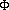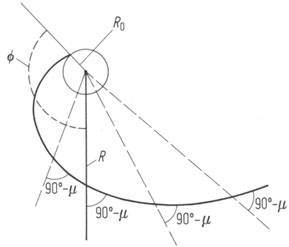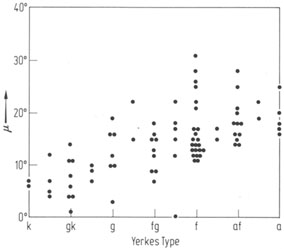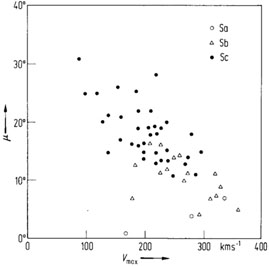## Spiral Structure

Reviews: [Galactic and Extragalactic Radio Astronomy (G.L. Verschuur, & K.I. Kellermann, eds.), Berlin, Springer (1988)].

The density-wave spirals are usually well represented by logarithmic spirals with only slowly changing pitch angle [Kennicutt, R.C. Jr. Astron. J. 86 (1981) 1847] (see Fig. 5):

R = R0 exp[x (R]   or   log R = A + Bwith x = tgµ(R);= winding angle; pitch angle µ being nearly constant. Pitch angles in different galaxies vary between a few and about 30°; the change of the pitch angle within any system is at most ±4° [Kennicutt, R.C. Jr. Astron. J. 86 (1981) 1847].

Material arms, on the other hand, possess a nearly hyperbolic form [Seiden, P.E., & Gerola, H. Astrophys. J. 233 (1979) 56]

R= const . Vrot(R) ,

dictated by the rotation curve of the galaxy.Figure 5. The logarithmic spiral.= winding angle; µ = pitch angle; 90° - µ = characteristic angle.

[Kennicutt, R.C. Jr. Astron. J. 86 (1981) 1847] investigated 113 objects and considered two functions: logarithmic spiral with constant pitch (i.e. linear in log R versus) and hyperbolic spiral (i.e. linear in Rversus R) with the result that neither logarithmic nor hyperbolic spirals accurately represent the spiral arms; but, within the limitations set by arm distortions, they may serve as adequate interpolation functions in normal spiral systems. The question of the mathematical form is irrelevant for the majority of real spiral arms.

Distribution of Pitch Angles:

1. Ryden, B., Astrophys. J. 396 (1992) 445.
2. Rand, R.J., Astrophys. J. 404 (1993) 593.Figure 6. The measured pitch angles vs. Yerkes-type galaxies [Kennicutt, R.C. Jr. Astron. J. 86 (1981) 1847].Figure 7. Correlation between pitch angle µ and maximum rotational velocity Vmax for spiral galaxies [Kennicutt, R.C. Jr. Astron. J. 86 (1981) 1847].

In strongly barred galaxies the arms are systematically distorted. Observational constraints of driving mechanism for spiral density waves: [Kormendy, J., & Norman, C.A. Astrophys. J. 233 (1979) 539].

Galaxy Bars and Resonances to Drive the Spiral Structure:

1. Berkhuijsen, E.M., Bajaja, E., & Beck, R., Astron. Astrophys. 279 (1993) 359.
2. Bertin, G., Pub. Astron. Soc. Pac. 105 (1993) 640.
3. Yuan, C., Pub. Astron. Soc. Pac. 105 (1993) 657.

Tidal Interaction as the Origin of Spiral Structure:

1. Sage, L.J., Astron. Astrophys. 272 (1993) 123.
2. Vader, J.P., Frogel, J.A., Terndrup, D.M., & Heisler, C.A., Astron. J. 106 (1993) 1743.

Main | Introduction | Basic Data | Galaxies | Cosmology | Particle Physics
Spectroscopy | Glossary and Lexicon of Term | Tabular Information
Graphical Relations | Annual Review Articles | Astrostatistics
CUP Monographs | Author Index | New Additions | Catalogs